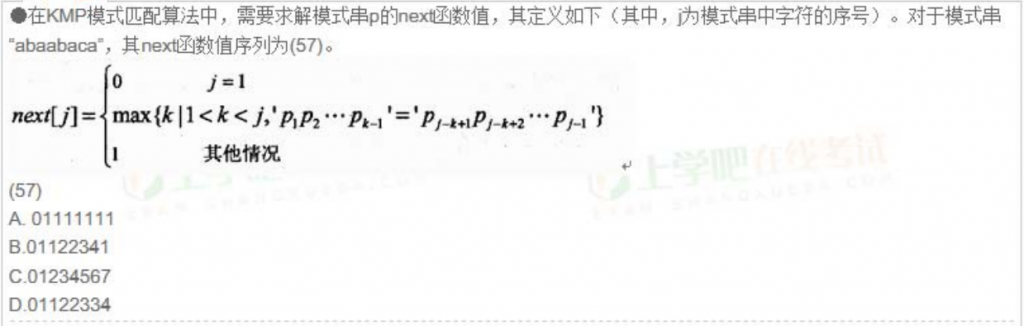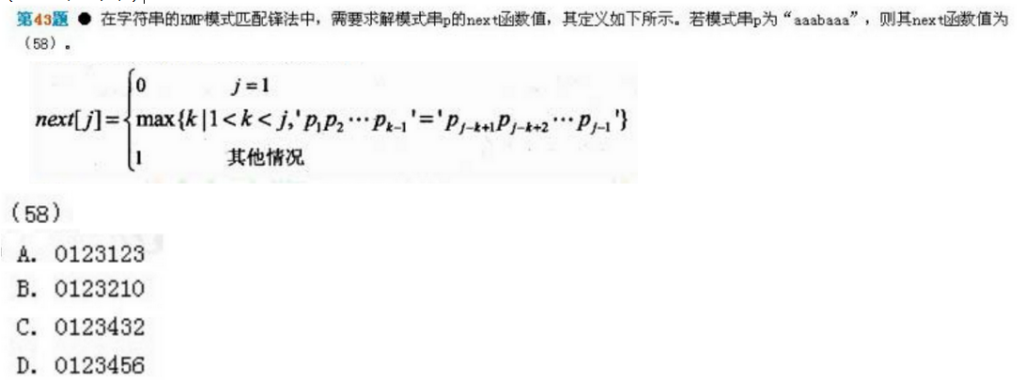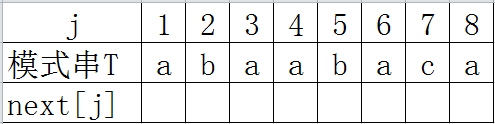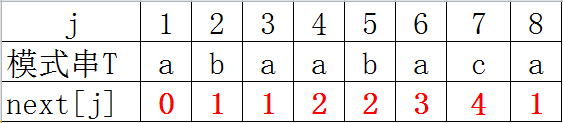# KMP算法Next数组计算

KMP算法是在最近这两年的软件设计师考试中才出现的。2次都是让求Next函数的序列（其实是）。先看看题吧。

（2011年下半年上午题）（2012年上半年上午题）j=1时， next=0

j=2时，k的取值为(1,j)的开区间，所以整数k是不存在的，那就是第三种情况， next=1

j=3时，k的取值为（1，3）的开区间，k从最大的开始取值，然后带入含p的式子中验证等式是否成立，不成立k取第二大的值。现在是k=2，将k导入p的式子中得，p1=p2，即“a”=“b”，显然不成立，舍去。k再取值就超出范围了，所以next不属于第二种情况，那就是第三种了，即 next=1

j=4时，k的取值为（1，4）的开区间，先取k=3，将k导入p的式子中得，p1p2=p2p3，不成立。 再取k=2，得p1=p3，成立。所以 next=2

j=5时，k的取值为（1，5）的开区间，先取k=4，将k导入p的式子中得，p1p2p3=p2p3p4，不成立。 再取k=3，得p1p2=p3p4，不成立。 再取k=2，得p1=p4，成立。所以 next=2

j=6时，k的取值为（1，6）的开区间，先取k=5，将k导入p的式子中得，p1p2p3p4=p2p3p4p5，不成立。 取k=4，得p1p2p3=p3p4p5，不成立。再取k=3，将k导入p的式子中得，p1p2=p4p5，成立。所以 next=3

j=7时，k的取值为（1，7）的开区间，先取k=6，将k导入p的式子中得，p1p2p3p4p5=p2p3p4p5p6，不成立。  再取k=5，得 p1p2p3p4=p3p4p5p6 ，不成立。 再取k=4，得 p1p2p3=p4p5p6 ，成立。所以 next=4

j=8时，k的取值为（1，8）的开区间， 先取k=7，将k导入p的式子中得，p1p2p3p4p5p6=p2p3p4p5p6p7，不成立。  再取k=6，得p1p2p3p4p5=p3p4p5p6p7，不成立。 再取k=5，得p1p2p3p4=p4p5p6p7，不成立。 再取k=4，得p1p2p3=p5p6p7，不成立。 再取k=3，得p1p2=p6p7，不成立。再取k=2，得p1=p7，不成立。k再取值就超出范围了，所以next不属于第二种情况，那就是第三种了，即 next=107-08
10-03171710-232万+
05-121万+
07-312万+
03-25
06-175568
01-271万+
08-13292
02-144940